# angles in quadrilateral

What size have angles in quadrilateral (4-gon) if they are in a ratio of 8: 9: 10: 13?

Result

a =  72 °
b =  81 °
c =  90 °
d =  117 °

#### Solution:Leave us a comment of example and its solution (i.e. if it is still somewhat unclear...):

Showing 0 comments:Be the first to comment!#### To solve this verbal math problem are needed these knowledge from mathematics:

Do you have a linear equation or system of equations and looking for its solution? Or do you have quadratic equation?

## Next similar examples:

1. 9-gon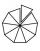Sum of interiol angles of 9-gon is:
2. Trapezoid v3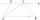The isosceles trapezoid has the angle at a firsst base 40 degrees less than the angle at second base. How large angles has this trapezoid ?
3. Find the 9Find the missing angle in the triangle and then name triangle. Angles are: 95, 2x+15, x+3
4. Fifth of the numberThe fifth of the number is by 24 less than that number. What is the number?
5. Obtuse angleWhich obtuse angle is creating clocks at 17:00?
6. The farmer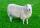The farmer had 140 sheep. For the next year, she decided to change the number of sheep in ratio 10: 7. How many sheep will he have then?
7. CandyPeter had a sachet of candy. He wanted to share with his friends. If he gave them 30 candies, he would have 62 candies. If he gave them 40 candies, he would miss 8 candies. How many friends did Peter have?
8. 1.5 divided1.5 divided by 1 = w divided by 4
9. Mother and daughter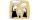The ratio of years mother and daughter is 5:2. After 7 years the ratio is 2: 1. How many years ago daughter was born?
10. Seven tractors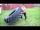Seven tractor plowed 308 ha field in four days. How many hectares of fields will plowe 9 tractors in 3 days at the same power?
11. Percentage reduction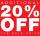Reducing the unknown number by 19% get number 700.7 Determine unknown number.
12. Roses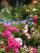On the large rosary was a third white, half red, yellow quarter and six pink. How many roses was in the rosary?
13. Apples 2How many apples were originally on the tree, if the first day fell one third, the second day quarter of the rest and on tree remained 45 apples?
14. EquationSolve the equation: 1/2-2/8 = 1/10; Write the result as a decimal number.
15. Unknown numberIdentify unknown number which 1/5 is 40 greater than one tenth of that number.
16. Simple equationSolve for x: 3(x + 2) = x - 18
17. Combine / add termCombine like terms 4c+c-7c